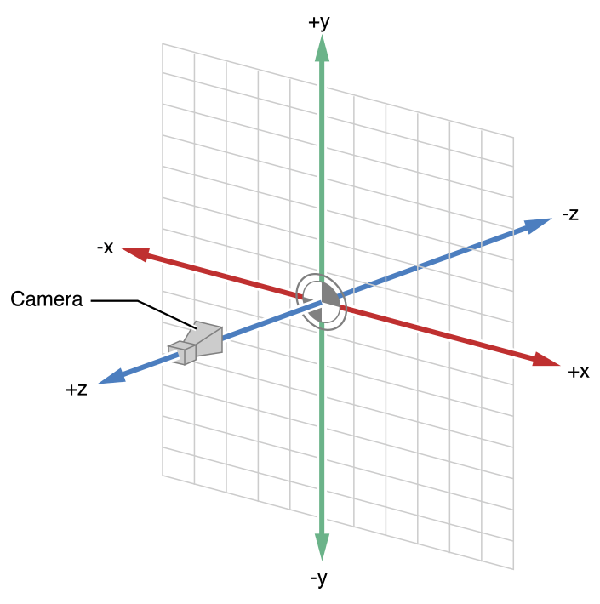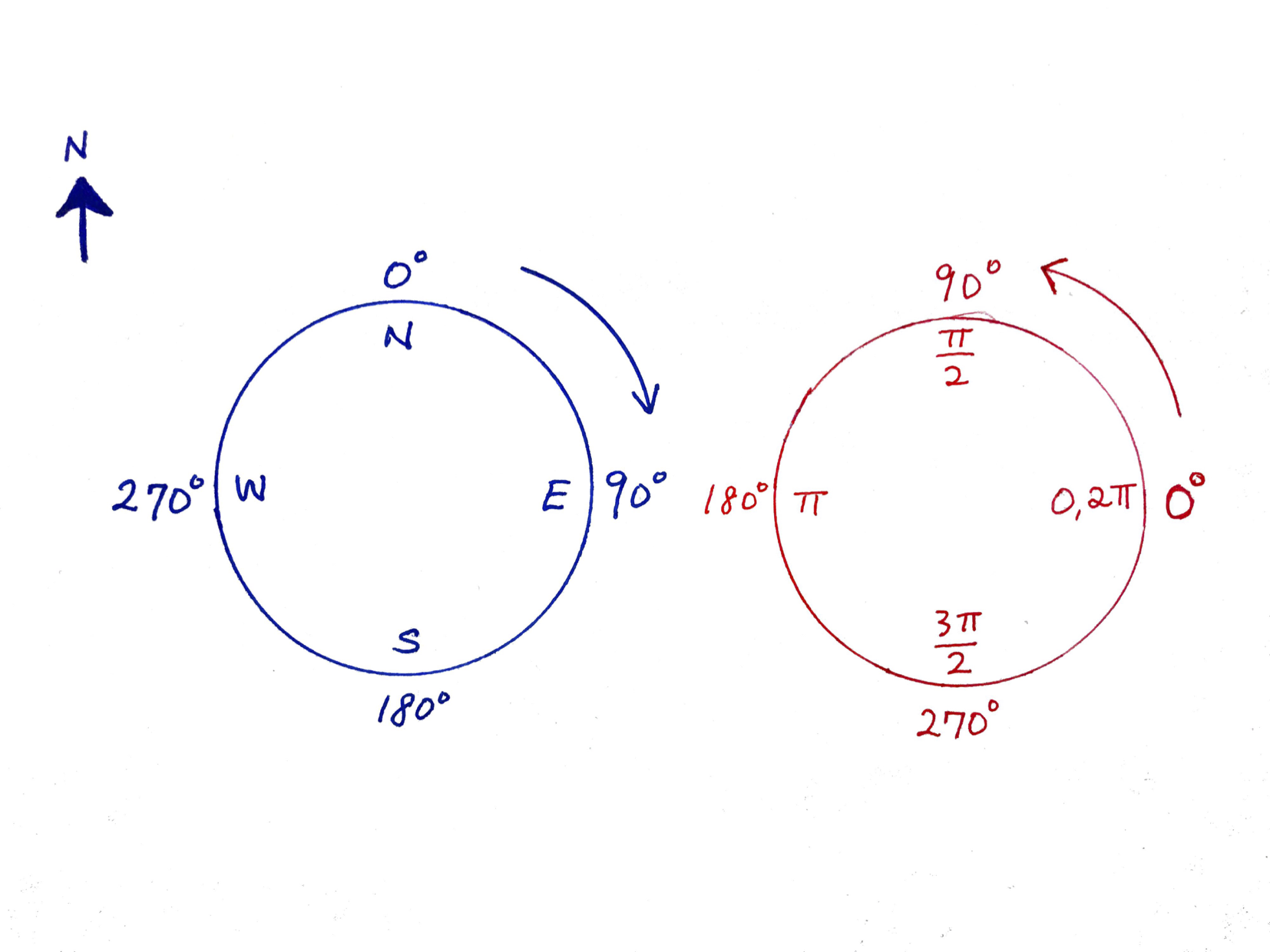# Converting Compass Degrees to Radians

Recently, I worked on an augmented reality (AR) iOS application where animated 3D models were placed directly in front of the user. The user’s heading was given in degrees, whereas the 3D models required radians for proper placement. However, the standard degrees-to-radians conversion formula of `degrees * pi / 180` seemed to place the 3D models incorrectly. After lots of head-scratching, I realized a subtle difference between degrees and radians that was the root of my issue.

##### A little iOS specific background info:

I retrieved the user’s heading (the direction in which the phone is pointed) in degrees via a `CLHeading` object from `CoreLocation`’s `CLLocationManager`. I then converted that value to radians, which was used to create a `SCNNode` with the 3D model at the given location using `(x: <dist>cos(rad), y: <model height>, z: <dist>sin(rad))`. This was just basic trigonometry, used against the SceneKit Coordinate System (shown below).However, as stated in the introduction, the models would never appear in the correct position.

#### Issue

The cause of this issue was actually very simple and straightforward once I drew a diagram:As you can see, compass degrees (left) start from North as 0, then go clockwise. On the other hand, radians (right) start from East as 0, then go counter-clockwise.

The standard conversion formula from degrees to radians uses the degrees listed on the right, whereas my heading value was given using the degrees listed on the left.

#### Solution

After some thought, I discovered the following formula, which converts from compass degrees to radian degrees:

``````(450 - compassDegrees) % 360 = radiansDegrees
``````

Turns out geometry class was pretty useful after all. 😅